# CBSE Worksheet for Chapter - 2 Algebra Class 8

## Find CBSE Worksheet for chapter-2 Algebra class 8

CLASS-8

BOARD: CBSE

Mathematic Worksheet - 2

TOPIC: Algebra

For other CBSE Worksheet for class 8 Mathematiccheck out main page of Physics Wallah.

#### Important Formulae:

1) (a+b) 2=a2+ 2ab+b2

2) (a-b) 2=a2- 2ab+b2

3) (a+b) 3=a3+ 3a2b+ 3ab2+b3=a3+b3 + 3ab (a+b)

4) (a-b) 3=a3- 3a2b+ 3ab2-b3=a3-b3 - 3ab (a-b)

5) (a3+b3) =(a+b) (a2-ab+b2)

6) (a3-b3) =(a-b) (a2+ab+b2)

7) (a2-b2) = (a+b) (a-b)

8) (a3+b3+c3- 3abc) = (a+b+c) (a2+b2+c2-ab-bc-ca)

9) (a3+b3+c3) = 3abc if a + b + c = 0

10) (a+b+c) 2=a2+b2+c2+ 2ab+ 2bc+ 2ca

Linear equation in one variable:

An equation involving a variable in the first degree is called a linear equation in one variable. It is of the form ax + b = 0,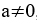x is variable.

x= - b/a is the root or solution of this equation.

An equation of the form ax2 + bx + c = 0, where a, b, c are constant and a 0, is called a quadratic equation in one variable ‘x’.

#### EXERCISE:

1) The factors of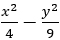are: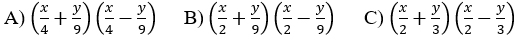D) None of these

2) In an examination a student was asked to find 3/14 th of a certain number. By mistake, he found 3/4 of it. His answer was 150 more than the correct answer. The number given to him is:

A) 290

B) 280

C) 240

D) 180

3) A man is 5 years older than his wife and the wife is now thrice as old as their daughter, who is 10 years old. How old was the man when his daughter was born?

A) 20 years

B) 23 years

C) 25 years

D) 30 years

4) If x + y=3 and xy=2, then the value of x3-y3 is equal to:

A) 6

B) 7

C) 8

D) 0

5) A farmer divides his herd of n cows among his four sons so that first son gets one half the herd, the second son gets one fourth, the third son gets one fifth, and the fourth son gets 7 cows, then n is:

A) 180

B) 140

C) 240

D) 100

6) If the difference of the squares of two numbers is 45, the square of the smaller number is 4 times the larger number, then the numbers are

A) 9, 6 or 9, -6

B) 5, 6 or 5, -6

C) 9, 5 or 9, -5

D) None of these

7) If 22x-y=32 and 2x+y=16 then x2+y2 is equal to:

A) 9

B) 10

C) 11

D) 13

8) The value of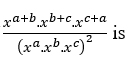is

A) x2

B) xa+b+c

C) xabc

D) x0

9) Ramu’s father is thrice old as Ramu. If father’s age is 45 years, then Ramu’s age is:

A) 45 years

B) 30 years

C) 15 years

D) 10 years

10) In a piggy bank the number of 25 paise coins are five times the number of 50 paise coins. If there are 1200 coins, find the amount in the bank?

A) Rs. 25

B) Rs.10

C) Rs.35

D) Rs.40

11) If quotient = 3x2 – 2x+ 1, remainder = 2x-5 and divisor = x+2, then the dividend is:

A) 3x3-4x2+x-3

B) 3x3-4x2-x+3

C) 3x3+4x2-x+3

D) 3x3+4x2-x-3

12) Solve for x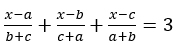:

A) ½(a+ b + c)

B) a +b + c

C) 3(a + b+ c)

D) 2(a b c)

13) The value of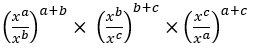is equal to:

A) 0

B) 1

C) x

D) x a+b+c

14) If p=3x+1, q= 1/3(9x+13) and p: q=6:5, then find x.

A) 7

B) - 7

C) 3

D) none of these

15) If (a2+b2)3= (a3+b3)2 then a/b + b/a =

A) 2/3

B) 3/2

C) 5/6

D) 6/5

16) If the expression x2 + K + 1/x2 is a perfect square, then the value of K is:

A) 2x

B) 2

C) 1

D) 1/2x

17) The value of x in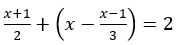is:

A) 1

B) 2

C) 3

D) 0

18) A number lying between 10 and 100 is seven times the sum of its digits. If 9 is subtracted from it the digits of the number are reversed. Then the number is:

A) 63

B) 54

C) 21

D) 42

19) The value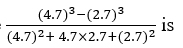is

A) 2

B) 7.4

C) 5

D) 84.14

20) If x=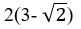, then is:

A) 2(3-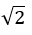)

B) 6- 2

C) 6 -D) 12

21) A person spends Rs.501 to buy tables and chairs. If the cost of each chairs is Rs. 15/- and tables cost 40% more than a chair and total number of articles bought was 27, how many tables and chairs did he buy?

A) 16 tables 11 chairs

B) 15 tables 12 chairs

C) 13 tables 14 chairs

D) 10 tables 17 chairs

1) (C)

2) (B)

3) (C)

4) (B)

5) (B)

6) (A)

7) (B)

8) (D)

9) (C)

10) (C)

11) (D)

12) (B)

13) (B)

14) (B)

15) (A)

16) (B)

17) (A)

18) (C)

19) (A)

20) (D)

21) (A)